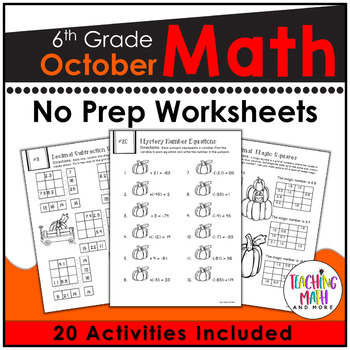## Wednesday, September 28, 2016

### Teaching Operations with Fractions {Grade 6}

I love teaching fractions to sixth graders despite the many obstacles that students might have to overcome. All students must master fractions to be able to master Algebra concepts later on. There are so many different ways to teach fractions from hands-on card sort activities, foldable notes, math stations, interactive notebook tools, and games. I've compiled a list of my favorite resources for engaging students in FRACTIONS.These dice games are intended to help students understand how to compare fractions, find equivalent fractions, add and subtract fractions with like and unlike denominators, apply and extend previous understandings of multiplication to multiply a fraction or whole number by a fraction, interpret and compute quotients of fractions and solve word problems involving division of fractions by fractions.
Included are:
-6 Different ROLL IT! NO PREP games
-2 Player games
-1 set of printable dice
-Each game is a Teacher facilitated activity for 15-30 minutes of classroom time
-NO PREP Print & GO!
-Topics Covered:
Comparing Fractions
Finding Equivalent Fractions
Subtracting Fractions
Multiplying Fractions
Dividing FractionsThese interactive notebook activities are intended to help students understand, interpret, and compute quotients of fractions and solve word problems involving division of fractions by fractions.
Included are:
-4 different Fractions activities to engage students
-Teacher facilitated activity for 60-90 minutes of classroom time
-Activities include: Vocabulary, Word Problems with Fractions, Comparing Fractions, and K.C.F. Method for Dividing Fractions
-Examples of Completed Interactive Math Notebook Activities are includedThis math station activity is intended to help students understand, interpret, and compute quotients of fractions and solve word problems involving division of fractions by fractions.
Included are:
-6 different stations to engage students
-Teacher facilitated activity for 60-90 minutes of classroom time
-Stations include:
1. Vocabulary
3. Practice of Fraction Operations
4. Additional Practice of Fraction Operations
5. Real World applications of Fractions
6. Word problems of Fraction Operations
-Student Station Guide helps students record their answersThis October Math NO PREP packet that will keep your sixth graders engaged! This packet is just plain fun. Not only is it PACKED with sixth-grade common core math problems, it also gives students fun coloring, puzzles, and problem solving. Use this packet for bellwork, classwork, extra credit, fast finishers, or homework!
THIS OCTOBER NO PREP MATH PACKET FEATURES: *20 different math puzzle worksheets
*FUN activities and puzzles centered on reviewing math curriculum.
*Packed with 6th grade common core math problems for review and practice.
*An ANSWER KEY for each worksheet.
*Lots of coloring fun. I’ve intentionally designed this packet with lots of pictures and borders to color. A MUST: Using a set of crayons or markers.
Topics Covered:
-Operations with Decimals
-Integers
-Factors & Multiples
-Rational Numbers
-Operations with Fractions
-Equations
-Updated for 2016-2017 School Year!

I hope these resources give you ideas for teaching operations with fractions. My favorite is the IMN activity where students have to "Keep it Change it Flip it" the fraction when dividing to find the answer. Students move the fractions in the interactive activity to come to the correct conclusions for the solution. Make learning fractions fun and engaging in your classroom too!